3．约束条件为等式的条件极值

求函数

y = f (x),  x=(x1,x2)

m(m<n)个约束条件

gk(x)=0,  k =1,2,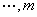[直接代入法]  从约束条件的m个方程中将其m个变量解出，用其余n-m个变量表示，然后直接代入函数中去，这样就变为一个求nm个变量的函数的无约束条件的极值问题.如果从约束方程能够将m个变量解出，这个方法是可行的.

[拉格朗日乘数法]  引进修正的系数

F=y+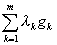i =1,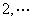,n

gk=0,   k =1,2,1  求函数

y =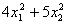2x1+3x2=6

由于

y =g =2x1+3x26=0

F = ()+λ(2x1+3x26)λ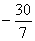x1=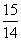,x2=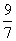x1=,    x2=D2=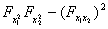=80>0

［惩罚函数法］ 在搜索极小点时引进修正函数

`F = y+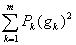(1)`

F = y+F = yF = y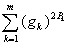F = y+P(g)2=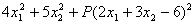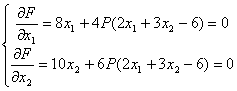x1=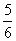x2x2=P很大时，x2趋于x1趋于，这就是稳定点.由于

D1==8(1+P)>0

D2==16(5+14P)>0